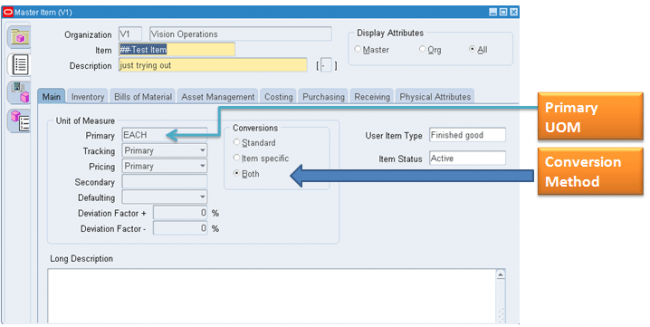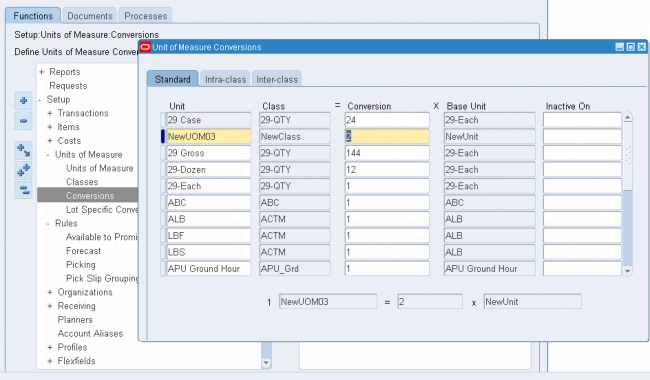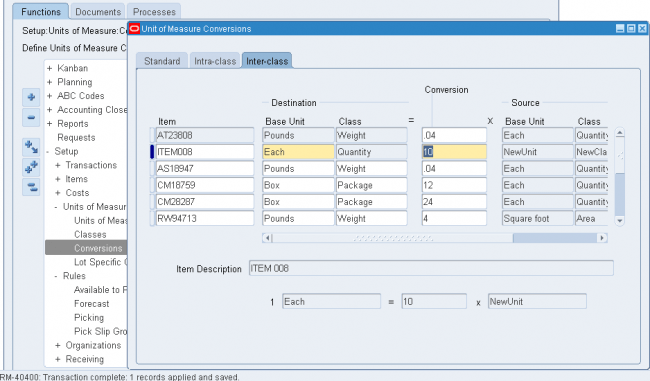# Defining Unit of Measure Conversions

Unit of measure conversions are numerical factors that enable you to perform transactions in units other than the primary unit of the item being transacted. You can define:
A conversion common to any item (Standard)
A conversion for a specific item within a unit of measure class (Intra-class)
A conversion for a specific item between unit of measure classes (Inter-class)
• Unit of measure conversions are not organization-specific.
• You must define a conversion between a non-base unit of measure and the base unit of measure before you can assign the non-base unit of measure to an item

Specifying Which Conversion to Use
When you define an item you decide which type of unit of measure conversion to use:

1. Itemspecific: Only uses unit of measure conversions unique to this item. If none exist, you can only transact this item in its primary unit of measure.
2. Standard: Uses standard unit of measure conversions for this item if an item-specific conversion is not available.
3. Both: Uses both item-specific and standard unit of measure conversions. If both exist for the same unit of measure and item combination, the item-specific conversion is used.Unit of Measure Conversions During Transactions
Whenever you enter an item’s quantity, the default is the primary unit of measure for the item. The list of values for the unit of measure field displays all units of measure for which you have defined standard and/or item-specific conversions from the primary unit of measure.
Transactions are performed in the unit of measure you specify. The conversion happens automatically and item quantities are updated in the primary unit of measure of the item.

Important
: Inventory transactions and on hand balance supports decimal precision to 5 digits after the decimal point. Oracle Work in Process supports decimal precision to 6 digits. Other Oracle Applications support different decimal precision. As a result of the decimal precision mismatch, transactions another Oracle Application passes may be rounded when processed by Inventory. If the transaction quantity is rounded to zero, Inventory does not process the transaction.
It is therefore suggested that the base unit of measure for an item is set up such that transaction quantities in the base unit of measure not require greater than 5 digits of decimal precision. Define a Standard conversion for any item
In standrad conversion you specify how one unit of mesaure is related to the primary unit of measure in the same class.
In standrad conversion you cant do the following
You cant specify any sort of intra class conversion
Relationship between two UOMs not invloving primary UOM

Navigate to the Unit of Measure Conversions window  & Select the Standard tabbed region.1 Enter a unit of measure.
2 Enter the conversion factor by which the unit of measure is equivalent to the base unit of measure established for this class.
For example, if one DZ (this unit of measure) is equivalent to 12 EA (base unit), the conversion factor is 12. Or, if EA is equal to one-twelfth of a DZ, the conversion factor is 0.08333. Define a conversion for a specific item within a unit of measure class (Intra-class)
We have seen in standard conversion we can only do convesrion from one UOM to primary UOM.
Suppose we have defined a UOM convsrion rule for a unit of measure UOM_XYZ as UOM_XYZ = 6 x Primary UOM but for a particular item the vonvsrion rate is not 6 in that case we define a intra class convesrion for  a particular item and when ever that item is transacted system ‘ll pick the convesrion rate from either intra class or standrad convesrion depending upon ITEM master set up.
Notes
We can do intra class conversion between two UOMs for a particular item only for a single class which is the UOM class of the primary UOM of the item.
For exampe Suppose ITEM001 has a primary UOM as Ea and UOM Ea belongs to class Quantity then we can define intra class conversion for the item ITEM001 between two UOMs in the class Quantity.
we cant define intra class convesrion between any other UOMs belonging to a differnt UOM class.Navigate to the Unit of Measure Conversions window.1. Select the Intra-class tabbed region.
2. Enter an item.
3. Enter a unit of measure.
4. Enter the conversion factor by which the unit of measure is equivalent to the base unit of measure established for this class.
For example, if one LB (this unit of measure) is equivalent to 16 OZ (base unit), the conversion factor is 16.

Define a conversion for a specific item between unit of measure classes (Inter-class)
Inter class conversion is used to convert an item from one primary UOM in one class to another primary UOM in a differnt class for a particular item. Navigate to the Unit of Measure Conversions window and Select the Inter-class tabbed region.1. Select an item.
2. Select the destination base unit of measure of the class to which you are converting a unit of measure.
3. Enter the conversion factor by which the source base unit is equivalent to the destination base unit.
For example, if one ML (source base unit) is equivalent to one GR (destination base unit), the conversion factor is one.
0 replies